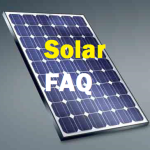# DIY Power Station – Part 8 – Calculations#### Watt’s Law Calculator

 Volts Amps Watts

Here is some information from the WindyNation website to help get started:

Volts-to-RPM Ratio
The Volts-to-RPM Ratio is one of the most important specifications to look out for in selecting your motor. Most DIYers use their motor to charge a 12-Volt battery, because of their cost and widespread availability. Charging a 12-Volt battery requires that the permanent magnet motor be generating at least 12 volts. If it doesn’t, then it can’t overcome the impedance of the 12V battery and the motor will never charge the battery. How do you know if your motor is capable of producing more than 12 volts when powered by the wind? Read on.

The volts-to-RPM ratio of a permanent magnet motor is defined as the volts required to spin the motor at a given RPM (rotations per minute). So let’s assume you have a permanent magnet motor that says the following on its label: “100 Volts, 2500 RPM.” This simply means if you supply the motor with 100 volts, it will spin at 2500 rpm. Its volts to RPM ratio is 0.040 V/RPM (100 divide by 2500).

### Volts to RPM Ratio Calculator

 Volts RPM V/RPM

This number provides a rough estimate of how many volts the motor will generate at a given rpm. Now let us assume our 100 Volt, 2500 rpm motor is spinning at 450 rpm. How much voltage will it produce at that rpm? The calculation is as follows:

(450 rpm) x (0.04 Volts/rpm) = 18 Volts

### Volts at RPM Calculator

 RPM V/RPM Volts

Now, there is one more step to do. We must multiply 18 Volts by 80%. Why? Because the 18 Volts is the number only if the motor is being used as a motor. This motor is not being used as a motor. It is being used as a generator, and it is not 100% efficient as a generator. It is roughly 80-85% efficient as a generator.

Therefore, 18 Volts x 0.8 = 14.4 Volts

### Efficiency Calculator

 Voltage PercentEfficiency Volts

We know how many Volts our motor will produce at 450 rpm: 14.4 volts. We must next consider the realistic RPM’s of a wind generator. You are most likely building a “small” wind generator that will be in the range of 100-500 Watts. Putting some well-constructed, 50-to-60 inch diameter blades on that motor will easily produce 450 rpm in wind speeds of 8-10 mph when the motor is under load (under load means the motor is connected to your battery bank. A generator has to work harder when it is under load and thus it will spin a little slower compared to when it is not under load). So this motor will begin charging a 12V battery bank in wind speeds of around 8-10 mph.

This is about what you are aiming for, and so we can conclude that this permanent magnet motor could work well for a wind generator.

A Volts-to-RPM ratio of AT LEAST 0.035 is the minimum requirement when looking for a permanent magnet motor. If the number is higher than 0.035 that is perfect. If the number is lower than 0.035, it will likely be insufficient unless it is located in an area with high winds.

Amperage Rating

The next item is the amperage rating of the motor. This provides information regarding how much current the motor will put out as a generator. From our experience, it is very difficult to predict what type of current your motor will put out as a generator. We’ve seen motors that expel more amps than that for which they are rated. However, one thing remains true: The higher the amperage rating, the better. You should be looking for a motor with a minimum amperage rating of at least 5 Amps. Anything above 5 Amps and you are good to go.

The power that a wind generator produces is directly proportional to the amps and voltage:

In fact, Power = Volts x Amperage

Remember, the more amps and volts the wind generator creates the more power it is producing!

So remember these three critical points:

Keep it simple: Only purchase a permanent magnet motor
Look for a minimum Volts to RPM ratio of 0.035
Look for a minimum amperage rating of 5